# Steps to Prepare Statement of Cash Flows

As we learned earlier, we can prepare a statement of cash flows using direct or indirect method. The two methods differ in terms of how the cash flow from operating activities is calculated. In the direct method, the cash flow from operating activities is computed directly as the net sum of all operating cash flows. In the indirect method, the operating cash flows are not directly reported. Instead you start with the net income taken from the income statement and then adjust it for the items that do not affect the cash flows.

As a general rule:

• Operating activities involve income statement items.
• Investing activities involve cash flows resulting from changes in investments and long-term asset items.
• Financing activities involve cash flows resulting from changes in long-term liability and stockholders' equity items.

To prepare the cash flow statement, we take the information from the income statement for the current year and balance sheets for the past two years. In the indirect method, the net income is adjusted for the deferrals and accruals to convert the accrual basis statement into a cash flow statement.

The first step in preparing the cash flow statement is to determine the net change in cash and cash equivalents. This forms our basis and the cash inflows and outflows in our cash flow statement will reconcile with this value. We will take the example of a fictional company called Always Ahead Inc. to demonstrate how to prepare the cash flow statement.

The next two pages show the Income Statement and Balance Sheet for Always Ahead Inc.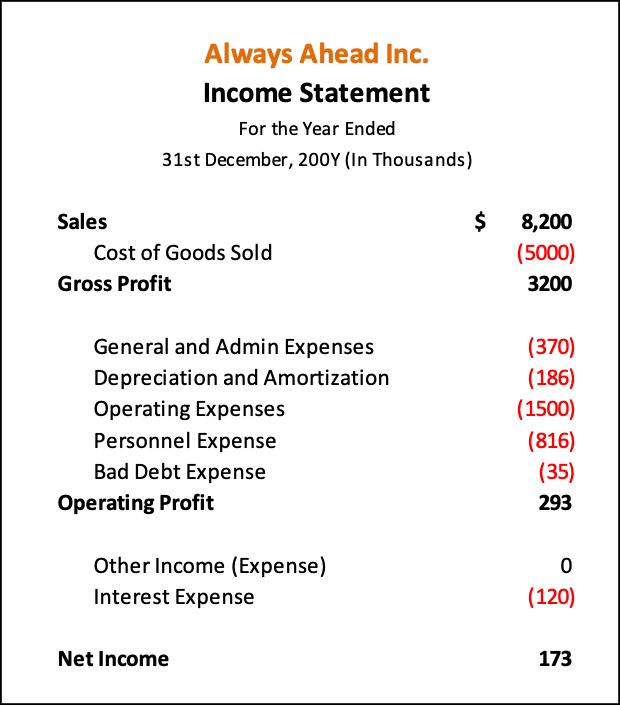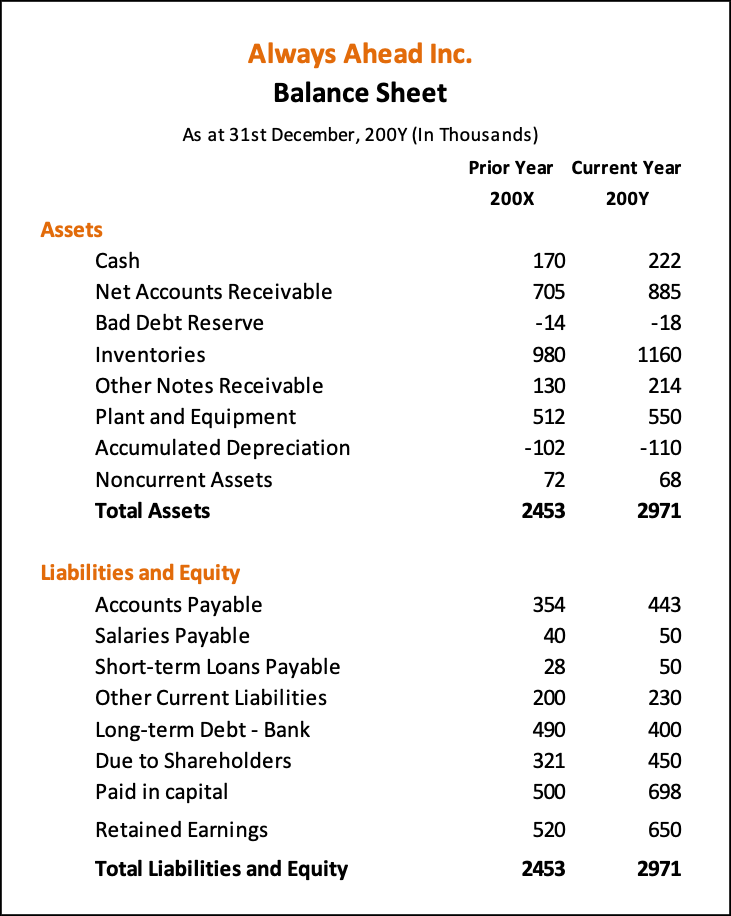The first step is to calculate the increase in cash balance from the balance sheet, which is $52,000 (222,000 – 170,000). Our cash flow statement should reconcile with this figure. The next step is to calculate the change in each balance sheet account. This is shown in the Balance Change column in the Balance Sheet table. For example, the accounts payable have increased by$89,000 (443,000 – 354,000). Similarly calculate the change in each balance sheet account.

The next step is to determine if the change in account balance is a cash inflow or a cash outflow, that is, whether, is a source or a use of cash. The following table summarizes what constitutes a cash inflow and what constitutes a cash outflow.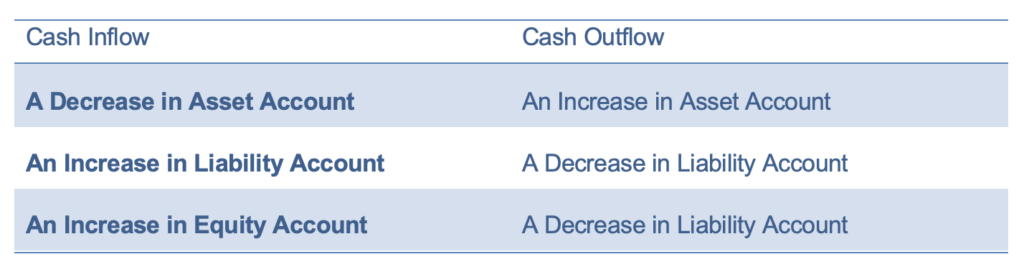We will also need to categorize each change in account balance as operating, investing or financing activity. The following guide will help you do that.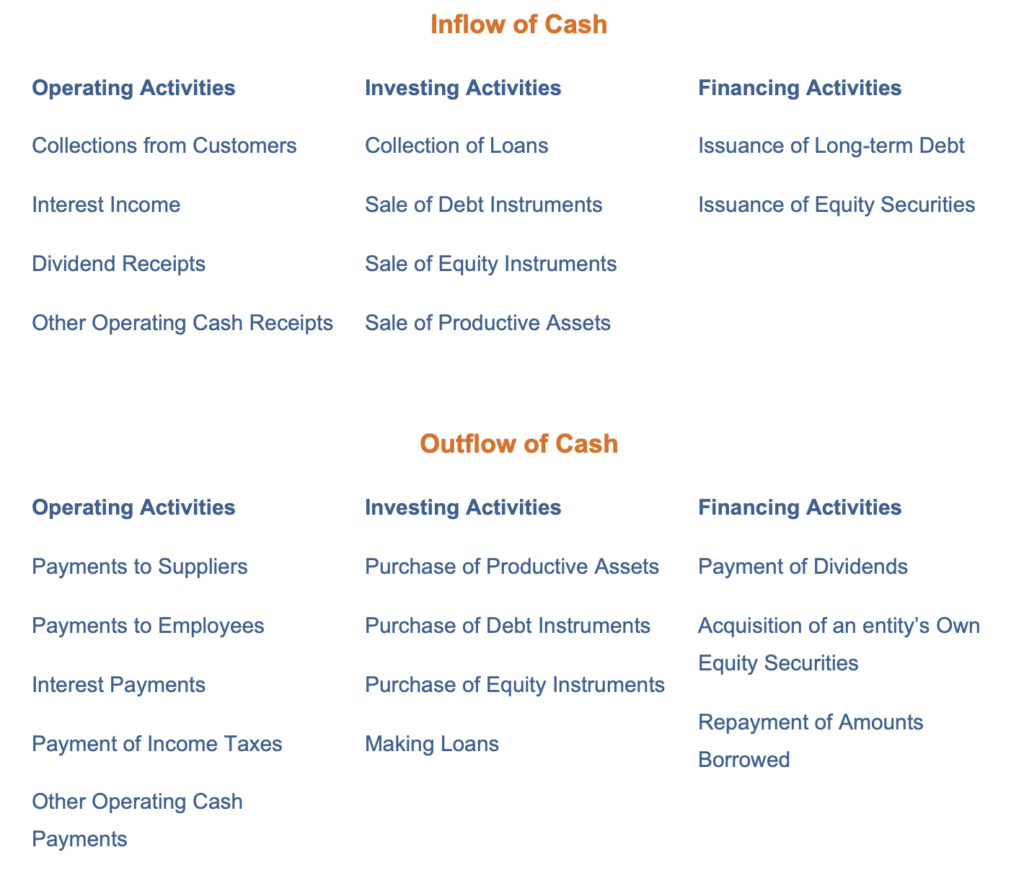Presented below is the cash flow worksheet created from the balance sheet that shows the balance change, cash source/use, and the activity type.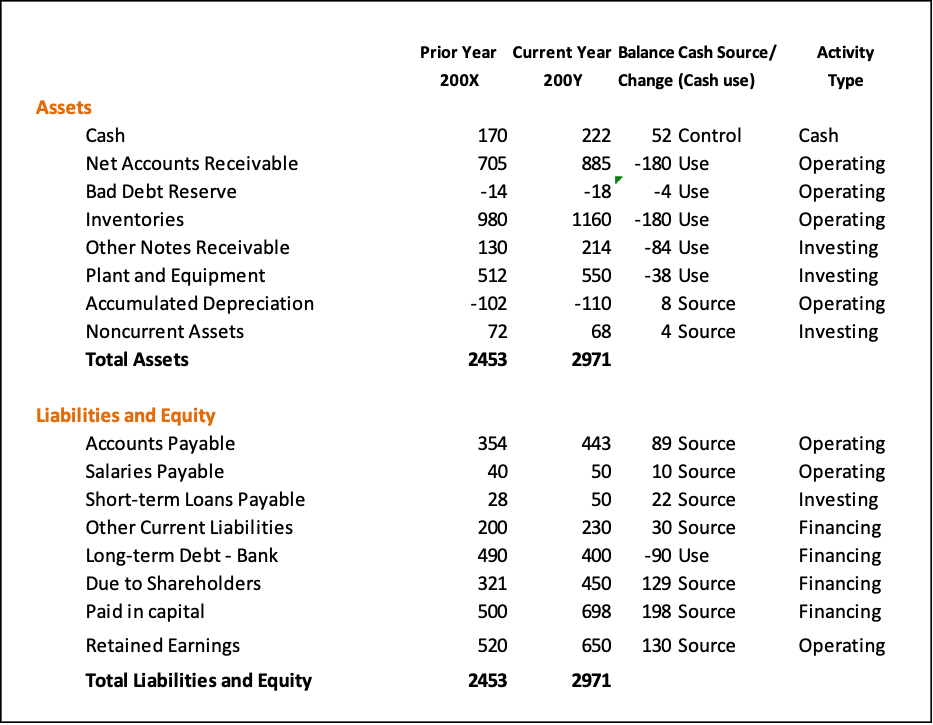We are now ready to construct the cash flow statement using either Direct or Indirect method. We will first calculate the cash flow from operating activities using both the methods. After that we will calculate the cash flow from investing and financing activities.

Get our Data Science for Finance Bundle for just $29$51.